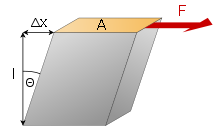# Shear Modulus Formula

A lateral deformation is observed in the object when a shear force is applied to it. The elastic coefficient is known as shear modulus of rigidity.  Shear modulus rigidity is the measurement of the rigidity of the object and it is obtained by measuring the ratio of shear stress of the object to the shear strain of the object.

## Shear Modulus Formula

 $$\begin{array}{l}G=\frac{Fl}{A\Delta x}\end{array}$$

### Notations Used In Shear Modulus Formula

• G is shear modulus in N.m-2
• F is the force acting on the body
• l is the initial length
• ∆x is the change in length
• A is the areaA shear modulus is applicable for the small deformation of the material by applying less shearing force which is capable to return to its original state. However not for the large sharing force because it results in permanent deformations of the object.

Example:

Shear modulus value for Steel is 7.9×1010

Shear modulus value for plywood is 6.2×108

From the above explanation, it is clear that steel is more rigid than plywood.

### Shear Modulus Unit And Dimension

 SI unit Pa Dimensional formula M1L-1T-2

### Solved Example:

Question 1: Compute the Shear modulus, if the stress experienced by a body is 5×104Nm2 and strain is 4×10-2.

Solution:

Given

Stress = 5×10Nm2

Strain =  4×10-2

ShearModulus (G) =Shear stress/Shear strain

ShearModulus (G) = (5×104)/ (4×10-2)

ShearModulus (G)  = 1.25×106Nm2

Stay tuned with BYJU’S to learn more on other Physics related concepts.